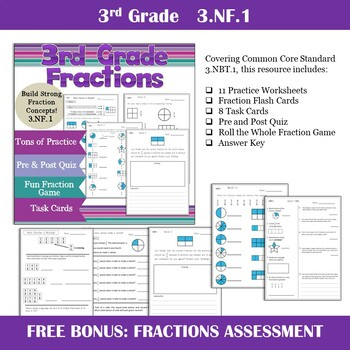DID YOU KNOW:
Seamlessly assign resources as digital activities

Learn how in 5 minutes with a tutorial resource. Try it Now

Learn More# Common Core Fractions Bundle Distance Learning3rd, Homeschool
Subjects
Standards
Resource Type
Formats Included
• Zip
•Activity
Pages
104 pages
\$14.00
Bundle
List Price:
\$19.96
You Save:
\$5.96
\$14.00
Bundle
List Price:
\$19.96
You Save:
\$5.96
Easel Activities Included
Some resources in this bundle include ready-to-use interactive activities that students can complete on any device. Easel by TpT is free to use! Learn more.

### Description

Bring fractions into the classroom with this bundle of 4 common core fraction units for 3rd grade. Each unit gives students a picture guide as well as practice. Detail answer key is included for each unit.

***Fractions on the Number Line (An introduction to using fractions on the number line)

***Fraction Frenzy (An introduction to fractions and a great intervention tool)

***Fraction Action (Reasoning and Comparing fractions to half)

***Fractions Beyond One (Working with Improper Fractions and Mixed Numbers)

***Roll the Whole (Fun game for exploring fractional parts)

***Flip Flop Fractions (Engaging game for comparing fractions)

***Mixed Number Bingo Cards

***Fraction Flash Cards (Includes a Sorting Activity)

Be the first to know about my new discounts, freebies and product launches: • Look for the green star near the top of any page within my store and click it to become a follower. You will then receive customized email updates about this store.

Total Pages
104 pages
Included
Teaching Duration
N/A
Report this Resource to TpT
Reported resources will be reviewed by our team. Report this resource to let us know if this resource violates TpT’s content guidelines.

### Standards

to see state-specific standards (only available in the US).
Compare two fractions with the same numerator or the same denominator by reasoning about their size. Recognize that comparisons are valid only when the two fractions refer to the same whole. Record the results of comparisons with the symbols >, =, or <, and justify the conclusions, e.g., by using a visual fraction model.
Express whole numbers as fractions, and recognize fractions that are equivalent to whole numbers. Examples: Express 3 in the form 3 = 3/1; recognize that 6/1 = 6; locate 4/4 and 1 at the same point of a number line diagram.
Recognize and generate simple equivalent fractions, (e.g., 1/2 = 2/4, 4/6 = 2/3). Explain why the fractions are equivalent, e.g., by using a visual fraction model.
Understand two fractions as equivalent (equal) if they are the same size, or the same point on a number line.
Explain equivalence of fractions in special cases, and compare fractions by reasoning about their size.